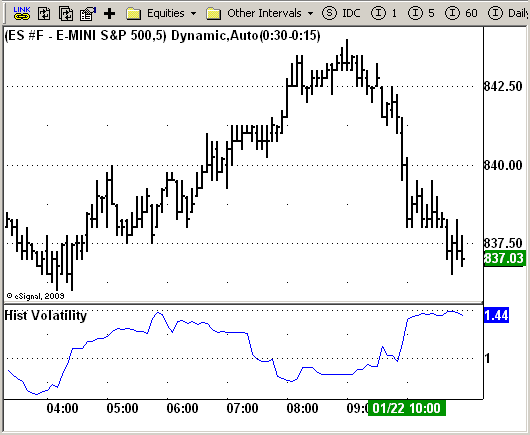# Historical Volatility

ICE Data Services -

 HistVolatility.efs EFSLibrary - Discussion Board

File Name: HistVolatility.efs

Description:
Historical Volatility

Formula Parameters:
LookBack : 20
Annual : 252

Notes:
Markets oscillate from periods of low volatility to high volatility
and back. The author`s research indicates that after periods of
extremely low volatility, volatility tends to increase and price
may move sharply. This increase in volatility tends to correlate
with the beginning of short- to intermediate-term moves in price.
They have found that we can identify which markets are about to make
such a move by measuring the historical volatility and the application
of pattern recognition.
The indicator is calculating as the standard deviation of day-to-day
logarithmic closing price changes expressed as an annualized percentage.`/*********************************Provided By: eSignal (Copyright c eSignal), a division of Interactive Data Corporation. 2008. All rights reserved. This sample eSignal Formula Script (EFS) is for educational purposes only and may be modified and saved under a new file name. eSignal is not responsible for the functionality once modified. eSignal reserves the right to modify and overwrite this EFS file with each new release.Description: Hist Volatility Version: 1.0 01/19/2009Formula Parameters: Default: LookBack 20 Annual 252Notes: Markets oscillate from periods of low volatility to high volatility and back. The author`s research indicates that after periods of extremely low volatility, volatility tends to increase and price may move sharply. This increase in volatility tends to correlate with the beginning of short- to intermediate-term moves in price. They have found that we can identify which markets are about to make such a move by measuring the historical volatility and the application of pattern recognition. The indicator is calculating as the standard deviation of day-to-day logarithmic closing price changes expressed as an annualized percentage.**********************************/var fpArray = new Array();var bInit = false;function preMain() { setPriceStudy(false); setStudyTitle("Hist Volatility"); setCursorLabelName("HisVol"); var x=0; fpArray[x] = new FunctionParameter("LookBack", FunctionParameter.NUMBER); with(fpArray[x++]){ setLowerLimit(1); setDefault(20); } fpArray[x] = new FunctionParameter("Annual", FunctionParameter.NUMBER); with(fpArray[x++]){ setLowerLimit(1); setDefault(252); }}var xPrice1 = null;var xPrice1Avg = null;var xStdDev = null;function main(LookBack, Annual) {var nState = getBarState(); if (nState == BARSTATE_ALLBARS) { if (LookBack == null) LookBack = 20; if (Annual == null) Annual = 252; } if ( bInit == false ) { xPrice1 = efsInternal("Calc_Price1"); xPrice1Avg = sma(LookBack, xPrice1); xStdDev = efsInternal("Calc_StdDev", LookBack, xPrice1Avg, xPrice1); bInit = true; } if (getCurrentBarCount() < LookBack + 1) return; var nAvg = xPrice1Avg.getValue(0); var HVol = xStdDev.getValue(0) * Math.sqrt(Annual); return HVol; }function Calc_Price1() {var nRes = 0; if (close(-1) == null) return; nRes = Math.log(close(0) / close(-1)); if (nRes == null) nRes = 1; return nRes;}function Calc_StdDev(LookBack, xPrice1Avg, xPrice1) {var nRes = 0; if (xPrice1Avg.getValue(0) == null) return; for (var i = 0; i < LookBack; i++) { nRes += (xPrice1.getValue(-i) - xPrice1Avg.getValue(0)) * (xPrice1.getValue(-i) - xPrice1Avg.getValue(0)); } nRes = Math.sqrt(nRes / LookBack); if (nRes == null) nRes = 1; return nRes;}`